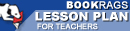Lesson Plans

# The Logic of Scientific Discovery Quiz | One Week Quiz A

This set of Lesson Plans consists of approximately 102 pages of tests, essay questions, lessons, and other teaching materials.
 View a FREE sampleName: _________________________ Period: ___________________

This quiz consists of 5 multiple choice and 5 short answer questions through Part II: Chapter 8, Probability.

## Multiple Choice Questions

1. What does Popper use to check his investigations?
(a) Assumptions.
(b) Universal statements.
(c) Testability.
(d) Mathematics.

2. What is a precondition for rational consideration?
(a) Subjectivity.
(b) Objectivity.
(c) Justification.
(d) Falsification.

3. How does Popper explain the differences in his selection process?
(a) Using discrete categories.
(b) Using letters.
(c) Using rational numbers.
(d) Using ordinal numbers.

4. Popper feels the principle of causality is not falsifiable and therefore regards it as what?
(a) Tautological.
(b) Empirical.
(c) Metaphysical.
(d) Physical.

5. What involves considering the number of favorable cases divided by the number of possible cases?
(a) Abstract probability.
(b) Numerical probability.
(c) Concrete probability.
(d) Non-numerical probability.

1. What is important to Popper's argument for a logic of science?

2. What does Popper feel is a fruitless exercise?

3. According to Popper, what philosophy ignores the hypothetical character of statistically derived estimates?

4. In order to validate his theory, what does Popper categorize regarding frequency theory?

5. What does the principle of causality say about all events?

 This section contains 192 words (approx. 1 page at 300 words per page) View a FREE sample